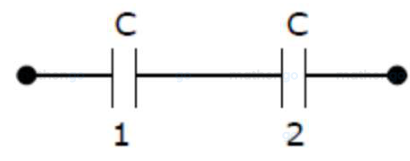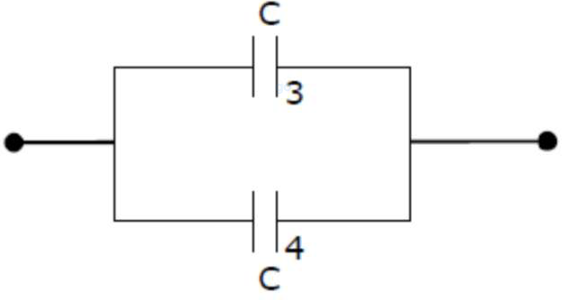# Two equal capacitors are first connected in series and then in parallel.

Question:

Two equal capacitors are first connected in series and then in parallel. The ratio of the equivalent capacities capacities in the two cases will be -

1. (1) $2: 1$

2. (2) $1: 4$

3. (3) $4: 1$

4. (4) $1: 2$

Correct Option: , 2

Solution:

(2)

Given that first connection$\Rightarrow \frac{1}{C_{12}}=\frac{1}{C}+\frac{1}{C} \Rightarrow C_{12}=\frac{C}{2}$

Second connection

$C_{34}=C+C=2 C$

Now, the ratio of equivalent capacities in the two cases will be

$\Rightarrow \frac{C_{12}}{C_{34}}=\frac{C / 2}{2 C} \Rightarrow \frac{C_{12}}{C_{34}}=\frac{1}{4}$